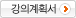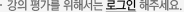## 주메뉴

### 고전역학

• 고려대학교
• 유종훈
• 주제분류
자연과학 >수학ㆍ물리ㆍ천문ㆍ지리 >물리학
• 강의학기
2016년 1학기
• 조회수
6,811
•
강의계획서Classical mechanics, together with classical electrodynamics and quantum mechanics, forms the core of present day theoretical training for undergraduate students. This course is the first class of an introduction to classical mechanics essential to understand most fields of physics.

Classical mechanics is the study of the motion of relatively large bodies in accordance with the general principles widely known as Newtonian mechanics. It has many important applications in the areas of astronomy, chemistry, biology, geology, and engineering. It describes the formation of the universe, the orbit of satellites, molecular collisions, stability of structures, dynamics of nonlinear systems, and etc.

The success in understanding this course is not only to work problems that exercise the principles but also to practice numerically the mechanical systems. It includes the dynamics of a particle, the harmonic oscillator, nonlinear oscillations, chaos, and gravitation.

Therefore, three or four problems at the end of each chapter are assigned to the homework every week. And one or two numerical calculations are given to practice the principles every week. In particular, we use the program of MATLAB to compute the problems in classical mechanics. The technical computing environment of MATLAB now provides an opportunity to easily solve realistic and more complicated problems. The following website is useful:

MATLAB: http://www.mathworks.com

#### 차시별 강의1Ch 1 Matrices, Vectors, and Vector Calculus 1 Introducton to vectors and vector calculus.2Ch 1 Matrices, Vectors, and Vector Calculus 1 Introduction to MATLAB Programming3Ch 2 Newtonian Mechanics Newton’s Laws4Ch 2 Newtonian Mechanics The equation of Motion for a particle5Ch 2 Newtonian Mechanics Energy6Ch 3 Oscillations Simple Harmonic Oscillator7Ch 3 Oscillations Damped Oscillations8Ch 3 Oscillations Oscillations by MATLAB Programming9Ch 4 Nonlinear Oscillations and Chaos Nonlinear Oscillations10Ch 4 Nonlinear Oscillations and Chaos Chaos11Ch 4 Nonlinear Oscillations and Chaos Nonlinear Oscillations by MATLAB Programming12Ch 5 Gravitation Gravitational Potential13Ch 5 Gravitation Ocean Tides14Ch 5 Gravitation Gravitation by MATLAB Programming15Ch 6 Euler’s Equation Methods in the Calculus of Variations#### 연관 자료#### 사용자 의견#### 이용방법

• 문서 자료 이용시 필요한 프로그램 [바로가기]

※ 강의별로 교수님의 사정에 따라 전체 차시 중 일부 차시만 공개되는 경우가 있으니 양해 부탁드립니다.